Latest Banking jobs   »

# Quantitative Aptitude Quiz For Bank of Baroda AO 2023 -17th May

Q1. Cost price of three type of laptop are in the ratio of 3 : 5 : 2 respectively. If the profit percent on three laptops are 25%, 10% and 20% respectively then find the overall profit percentage is?
(a) 19%
(b) 22%
(c) 15%
(d) 16.5%
(e) 25%

Q2. A shopkeeper sold an article at a profit of 1/8 th of its SP to a person. If shopkeeper sold it at 25% more than previous selling price it will have Rs 68 more as profit. Find its selling price.
(a) Rs. 280
(b) Rs. 272
(c) Rs. 290
(d) Rs. 300
(e) Rs. 264

Q3. The radius of a cylinder & a sphere is same, and ratio of height and radius of cylinder is 2 : 1.If the volume of sphere is 288 π cm³ then find the volume of cylinder? (in cm³)
(a) 438 π
(b) 426 π
(c) 420 π
(d) 432 π
(e) 444 π

Q4.A right circular cone and a right cylinder have a circle of the same radius as their base and their heights are equal to the radius itself. If a hemisphere has the same radius, then their volumes are in the proportion of
(a)2:3:1
(b)1:3:2
(c)1:2:3
(d) 1:3:1
(e)1:1:3

Q5. If ratio of area of a circle to the area of an equilateral triangle is 16π:√3, then what is the ratio of radius of the circle to the length of the side of equilateral triangle?
(a) 2: 3
(b) 2: 1
(c)1: 2
(d) 3: 2
(e) 4:3

Q6. In how many ways the word ‘INTICINCO’ can be written so that ‘T’ always comes at end.
(a) 720
(b) 1680
(c) 5040
(d) 1024
(e) 210

Q7. If three coins are tossed simultaneously, find the probability of getting minimum 2 head at a time.
(a) 1/2
(b) 3/8
(c) 5/8
(d) 3/4
(e) 7/8

Q8. A sells man has 6 red and 5 black shirts. If two shirts are picked up randomly, then what is the probability of getting shirts of same color?
(a) 6/11
(b) 5/11
(c) 10/11
(d) 7/11
(e) 4/11

Q9. A box contains 12 red, 6 green and ‘x’ yellow balls. Probability of choosing one green ball out of the box is 2/9, then find the probability of choosing one ball which can be either red or yellow?
(a) 4/9
(b) 5/9
(c) 2/3
(d) 7/9
(e) 8/9

Q10. A person brought some articles and sold 60% of them for the price he paid for total articles. He sold the remaining articles at 10% profit. Find his total profit percentage.
(a) 50%
(b) 46%
(c) 44%
(d) 10%
(e) 56%

Solutions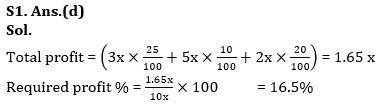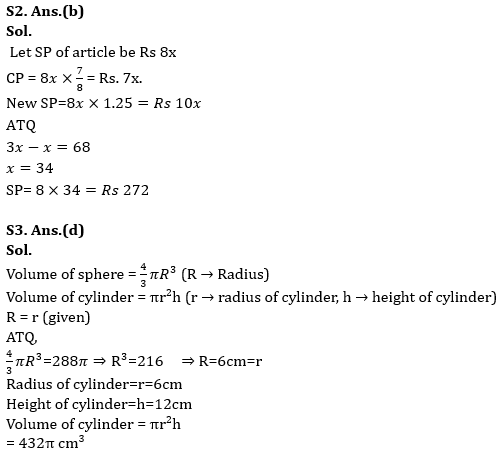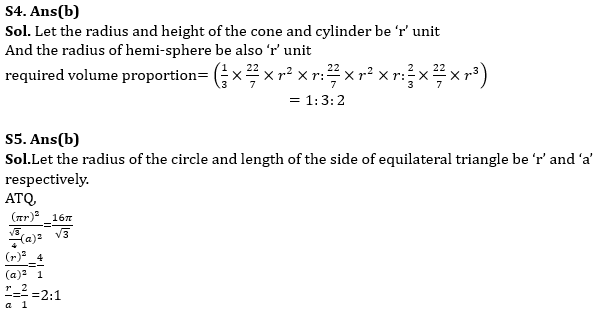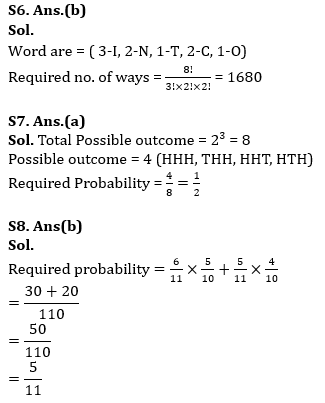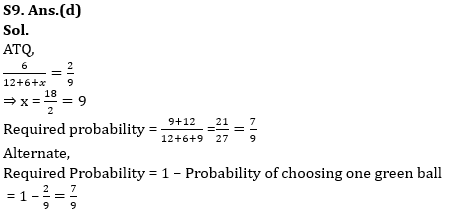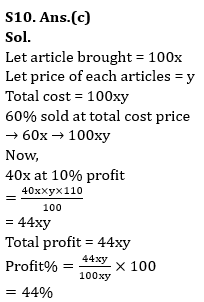## FAQs

### What is the selection process of Bank of Baroda AO 2023?

The selection process of Bank of Baroda AO consists of Online exam and Interview

#### Congratulations!Union Budget 2023-24: Free PDF